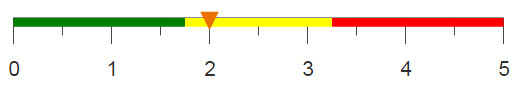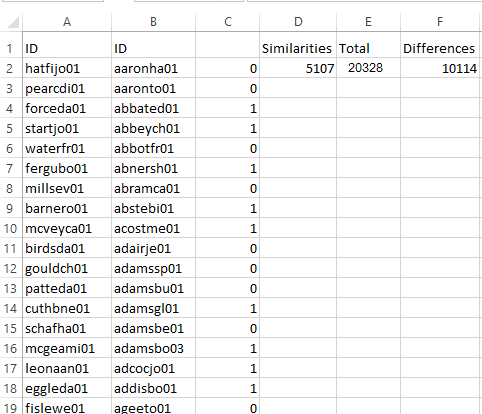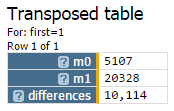# Determining the differences between two data sets

You can use 1010data to determine the number of differences between two tables, similar to using COUNTIF in Excel.

## Difficulty## Objective

You have two tables and you would like to know how many points differ between the two data sets. You know how to do this in Excel by using COUNTIF and SUM, and you would like to produce the same results using 1010data. For this analogue, the number of differences between the players in the Batting table and the Fielding table are calculated.

## Excel solution

After importing the two tables with all duplicates removed into Excel, the two columns of player ID's are placed side by side in the same worksheet. This allows the comparisons to take place. Then the COUNTIF function is used to compare if any single entry is contained in both columns. SUM then adds these results to determine the number of similarities between the tables.

`=COUNTIF(\$B:\$B,\$A2)`
`=SUM(C:C)`

Another COUNTIF function is used to count the total number of players.

`=COUNTIF(A:B,"*")-2`

Then finally, the number of differences are calculated by subtracting twice the similarities from the total count.

`=E2-2*D2`

An image of the Excel worksheet can be seen below.Calculating these results in Excel is not ideal. Pre-adjustments to the tables are required before you can begin work in Excel. In addition, the time to complete the comparison between the two ranges is lengthy. With 1010data, you can complete all parts of the calculation in one place and at a faster speed.

## 1010data Macro Language solution

```<base table="pub.demo.baseball.batting"/>
<willbe name="first" value="g_first1(lahmanid;;lahmanid)"/>
<sel value="first=1"/>
<colord cols="lahmanid"/>
<merge table2="pub.demo.baseball.fielding" type="intersect">
<willbe name="first" value="g_first1(lahmanid;;lahmanid)"/>
<sel value="first=1"/>
<colord cols="lahmanid"/>
</merge>
<tabu label="Number of Rows">
<tcol source="lahmanid" fun="cnt" label="Count"/>
</tabu>
<merge table2="pub.demo.baseball.batting">
<willbe name="first" value="g_first1(lahmanid;;lahmanid)"/>
<sel value="first=1"/>
<merge table2="pub.demo.baseball.fielding">
<willbe name="first" value="g_first1(lahmanid;;lahmanid)"/>
<sel value="first=1"/>
</merge>
<tabu label="Number of Rows">
<tcol source="lahmanid" fun="cnt" label="Count"/>
</tabu>
</merge>
<transpose/>
<willbe name="differences" value="m1-2*m0"/>```

With 1010data, tabulations are utilized to determine the number of rows, and therefore the number of players.

In the Batting table, a column is created using <willbe> to distinguish the first occurrence of every player with the function g_first1(G;S;O). A selection statement then selects only this first occurrence to eliminate duplicates. Since you are only interested in the differences of players, <colord> is used to show only the lahmanid column.

Then, the Fielding table is merged with the Batting table, with the same transformations applied. A key step here is using type="intersect" with the merge, therefore, only players that appear in both tables will appear in the new merged table. Now that you have a table that contains only the players the two tables have in common, you can count them by performing a tabulation and using fun="cnt".

The total number of players contained in both tables is needed to then determine the number of differences. This is done by again applying the same transformations to each individual table and merging them together. Then the count tabulation is again used to count the total number of players. Additionally, all of this is done inside another merge, so the results can be combined with the results of the previous tabulation.

Due to the nature of the table, a <transpose/> operation is needed to allow both results to be visible. Finally, another <willbe> column is created where its value is equal to the total number of players less twice the similarities. The similarities need to be doubled to account for their presence in both tables. Below is an image of your final table.## Further reading

If you would like to learn more about the functions and operations discussed in this recipe, click on the links below:

g_first1(G;S;O)

<transpose/>

<link>